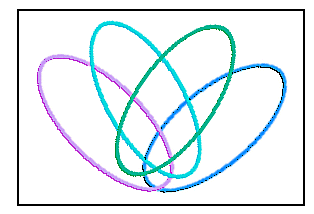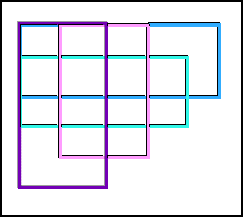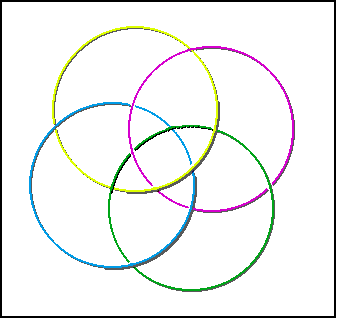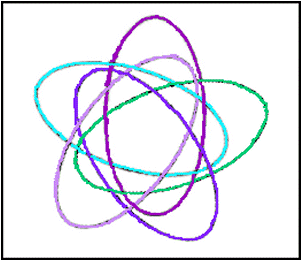#### The Venn diagram shows four sets, A, B, C, and D. Each of the sixteen regions represents the intersection over a subset of {A, B, C, D}. Can you find the intersection of all four sets? The tree diagram below displays the sixteen possible subsets. Try to match each of the subsets with a region in the diagram. (Check your answers)#### Here are two more Venn diagrams with four sets#### Do you know why this is not a Venn Diagram for 4 sets?